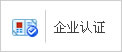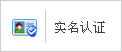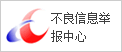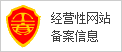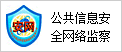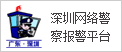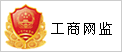P0502 逻辑表达式

P0502逻辑函数的代数化简法

L=AC+CD

L=AC+CD        与一或表达式

=AC・CD       与非一与非表达式

=(A+C)(C+D)   或一与表达式

=(A+C)+(C+D)   或非一或非表达式

=AC+CD      与一或非表达式L1=A BC+ABC

L2=A(BC+BC)+A(BC+BC)

L2 =ABC+ABC+AB C+A BC

=AB(C+C)+AB(C+C)

=A(B+B)=A相关IC型号推荐技术资料